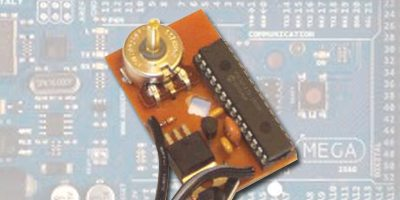# DC Motor Control Using PWM

STUTEE SAXENA

9890Small DC motors are efficiently controlled using pulse-width modulation (PWM) method. The circuit described here is built around an LM324 low-power quad-operational amplifier. Of the four op-amps (operational amplifiers) available in this IC, two are used for triangular wave generator and one for comparator.

Op-amp N2 generates a 1.6kHz square wave, while op-amp N1 is configured as an integrator. The square wave output of N2 at its pin 14 is fed to the inverting input (pin 2) of N1 through resistor R1. As N1 is configured as an integrator, it outputs a triangular wave of the same frequency as the square wave. The triangular wave is fed to pin 5 of op-amp N3, which is configured as a comparator.The reference voltage at pin 6 of the comparator is fixed through the potential divider arrangement formed by potmeter VR1 and resistors R4 and R5. It can be set from –6V (lowermost position of VR1) to +6V (uppermost position of VR1).

The triangular wave applied at pin 5 of N3 is compared with the reference voltage at its pin 6. The output at pin 7 is about +12V when the voltage at pin 5 is greater than the voltage at pin 6. Similarly, the output at pin 7 is about -12V when the voltage at pin 5 is lower than the voltage at pin 6.

The output from comparator N3 is the gate voltage for n-channel MOSFET (T1). T1 switches on when the gate voltage is positive and switches off when the gate voltage is negative. Setting of the reference voltage therefore controls the pulse-width of the motor.

When T1 is switched on for a longer period, the pulse width will be wider, which means more average DC component and faster speed of the motor. Speed will be low when the pulse width is small. Thus potmeter VR1 controls the speed of the motor.

Assemble the circuit on a general-purpose PCB and enclose in a suitable cabinet. The circuit requires ±12V power supply for its working. It can also be modified to control the speed of a 6V or 24V DC motor.